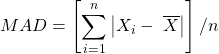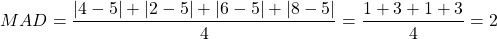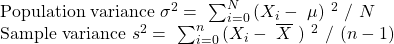Concept 7: Measures of Dispersion | IFT World
101 Concepts for the Level I Exam

# Concept 7: Measures of DispersionRange is the difference between the maximum and minimum values in a data set.

Range = maximum value – minimum value

The annual returns of a portfolio manager for the past 4 years are 4%, 2%, 6%, 8%. Calculate the range.

Solution:

Range = 8% – 2% = 6%

Mean absolute deviation (MAD) is the average of the absolute values of deviations from the mean.The annual returns of a portfolio manager for the past 4 years are 4%, 2%, 6%, 8%. Calculate MAD.

Solution:

X = (4 + 2 + 6 + 8)/4 = 5Variance is defined as the mean of the squared deviations from the arithmetic mean.Standard deviation is the positive square root of the variance. It is often used as a measure of risk.

The annual returns of a portfolio manager for the past 4 years are 4%, 2%, 6%, 8%. Calculate the population and sample standard deviations.

Solution:

It is advisable to use the financial calculator instead of the formula on the exams. The keystrokes for the above example are: [2nd] [DATA], [2nd] [CLR WRK], 4 [ENTER], [↓] [↓] 2 [ENTER], [↓] [↓] 6 [ENTER], [↓] [↓] 8 [ENTER], [2nd] [STAT] [ENTER], [2nd] [SET] press repeatedly till you see 1-V. Keep pressing [↓] to see the following values: N = 4, X = 5, Sx = 2.58, σx = 2.24.

Note: If you are asked to calculate the variances, simply square the standard deviations.

LIVE WEEKEND CLASSES *For 2024 Exam candidates*
This is default text for notification bar